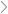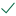Questions & AnswersStatistics and ProbabilityA leading magazine (like Barron's) reported at one time that the...

QuestionAnswered step-by-step

# A leading magazine (like Barron's) reported at one time that the...

A leading magazine (like Barron's) reported at one time that the average number of weeks an individual is unemployed is 30 weeks. Assume that the length of unemployment is normally distributed with population mean of 30 weeks and the population standard deviation of 7 weeks. Suppose you would like to select a random sample of 10 unemployed individuals for a follow-up study. Round the answers of following questions to 4 decimal places.

a. What is the distribution of X? X ~ N(___,____)

b. What is the distribution of ¯xx ~ N(____,_____)

c. What is the probability that one randomly selected individual found a job less than 31 weeks?

d, For 10 unemployed individuals, find the probability that the average time that they found the next job is less than 31 weeks?

e. For part d), is the assumption of normal necessary? Yes or NoSolved by verified expert10,000 step-by-step explanations